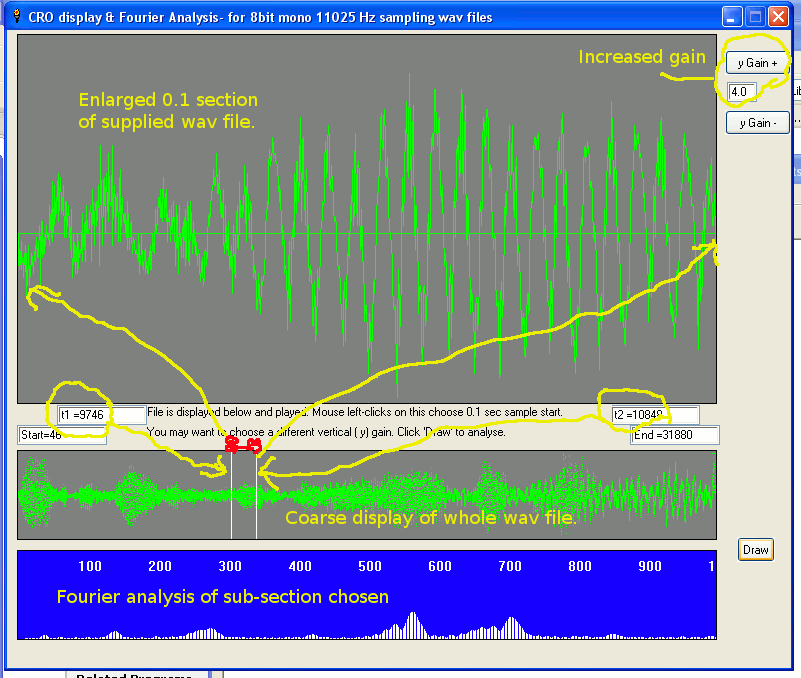# Display & Fourier analysis of a wav fileThis program displays a supplied 8 bit mono 11025 Hz sampled wav file in both time and frequency domain.
It could easily be modified to read other wav file formats.
I recommend the NCH software for creating sound samples- eg pure tones, sweeps, etc- and converting them between formats.
In 'time domain' we simply draw the sound amplitude as a graph against frequency. It looks like a classic 'oscilloscope'. Built my first, valve & crt based, in about 1965. Nowadays I'd use data-grabbing software.
In 'frequency domain' we are interested in which frequencies appear in a chosen section of a wave, and with what amplitude. My program implements this in BASIC, using the definition of a Fourier Analysis. At each frequency of interest, the wav amplitude is multiplied at each sample time point by the value of the sine wave frequency at that same time, and the total of these products is accumulated. This gives for each frequency a number showing how much of that frequency sin wave was contained. You do this again for the 90 degree shifted cosine components. The result is a graph of how much of each frequency there was in the sample.

In BASIC the multiple loops take ages. It can be shortened ( the 'Fast Fourier Transform') but then it is harder to see what is happening. More practically, you'd use a pre-compiled fft dll ( the 'Fastest Fourier Transform in the West'- fftw.dll.) However, programming the transform in terms of its definition, and applying it to real waves, is a great way to get real understanding.

The Fourier Synthesis ( putting together a wave from a description of how much of each frequency to include) is very easy, and also fun.

Fourier transformation is a magic idea and well worth investigating for its implications and applications in sound, images, etc.